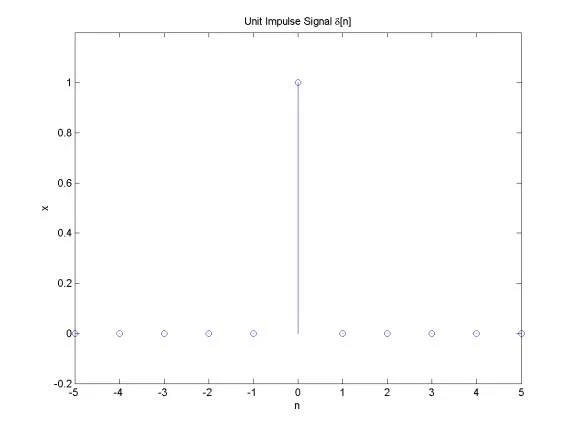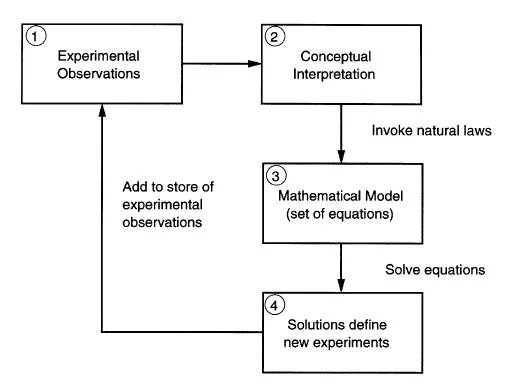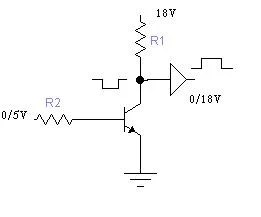## What is a linear system?Discrete system is nothing more than algorithm, where input is transformed to output. The output is transformed by operator L() which describes discrete system. Lets see few most common operators of discrete systems. Delay This means that output queue is delayed by on sample. Multiplication This operator takes each sample of input queue and multiplies by constant a. Sum operator Takes two or more sample queues and adds them in the output. Assuming we can say, that the system is linear if input sum reaction is equal to sum of inputs reactions: The system has stable parameters if: y(n-k)=L(x(n-k)), this means that output delay should be the same as input. It is obvious that delay, multiply and sum operators are linear and has stable parameters. We will need then for further lessons.

## Understanding of discrete signalsDiscrete signals can be generated by software or obtained from real world through ADC. Discrete signals are sampled from analog signals. So you get samples in fixed time intervals. Discrete signal is as sequence of numbers. The element number n of sequence is marked as x(n). The most common number rows: Unit sample sequence d[n] = 1, if n=0 d[n] = 0, otherwise You can describe it in Matlab like % Plot an unit impulse signal n = -5:5; x = 0*n; index=find(n==0); x(index)=1; % plot stem(n, x); axis([-inf, inf, -0.2, 1.2]); xlabel(‘n’); ylabel(‘x’); title(‘Unit Impulse Signal delta[n]’); Unit Step Sequence u[n] = 1, if n>=0 u[n] = 0, otherwise You can describe it in Matlab like: % Plot an unit impulse signal n = -5:5; x = 0*n; index=find(n>=0); x(index)=1; % plot stem(n, x); axis([-inf, inf, -0.2, 1.2]); xlabel(‘n’); ylabel(‘x’); title(‘Unit Step Signal u[n]’); As you can see step is nothing more than set of impulses. And impulse can be expressed as d[n]=u(n)-u(n-1); Thus any sequence of numbers can be expressed asset of impulses like this: For example sin() sequence can be written like this: Matlab script would look like this: % Plot a sinusoidal signal n = 0:40;…

The AD9288 is a dual 8-bit monolithic sampling analog-to-digital converter with on-chip track-and-hold circuits and is optimized for low cost, low power, small size and ease of use. The product operates at a 100 MSPS conversion rate with outstanding dynamic performance over its full operating range. Each channel can be operated independently. The ADC requires only a single 3 V (2.7 V to 3.6 V) power supply and an encode clock for full-performance operation. No external reference or driver components are required for many applications. The digital outputs are TTL/CMOS compatible and a separate output power supply pin supports interfacing with 3.3 V or 2.5 V logic. The encode input is TTL/CMOS compatible and the 8-bit digital outputs can be operated from +3 V (2.5 V to 3.6 V) supplies. User selectable options are available to offer a combination of power down modes, digital data formats and digital data timing schemes. In power-down mode, the digital outputs are driven to a high-impedance state. Fabricated on an advanced CMOS process, the AD9288 is available in a 48-pin surface mount plastic package (7×7 mm, 1.4 mm 48-Pin LQFP) specified over the industrial temperature range (-40Â°C to +85Â°C). Pin out designed for 10-bit…

## LPC229x protel library

The LPC2292 microcontrollers are based on a 16-bit/32-bit ARM7TDMI-S CPU with real-time emulation and embedded trace support, together with 256 kB of embedded high-speed flash memory. A 128-bit wide memory interface and a unique accelerator architecture enable 32-bit code execution at the maximum clock rate. For critical code size applications, the alternative 16-bit Thumb mode reduces code by more than 30 pct. with minimal performance penalty. With their 144-pin LQFP144 package, low power consumption, various 32-bit timers, 8-channel 10-bit ADC, 2/4 (LPC2292) advanced CAN channels, PWM channels and up to nine external interrupt pins these microcontrollers are particularly suitable for automotive and industrial control applications as well as medical systems and fault-tolerant maintenance buses. The number of available GPIOs ranges from 76 (with external memory) through 112 (single-chip). With a wide range of additional serial communications interfaces, they are also suited for communication gateways and protocol converters as well as many other general-purpose applications. Here you can try my generated LPC2292 protel library. Also this is same Protel library for all LPC229x microcontrollers.Â LPC2292 protel library

## Emergency supply source for your electronic deviceSometimes you are needed to maintain power supply for your device for some time when the main source fails. It can be alarm system or some light source or digital clock. Of course if source interrupts are very short, there can be capacitor with big capacity connected, but it is not reliable. Better use backup battery and diode. This solution is very simple and cheap. The diode protects the back current float to battery. This doesn’t take much space to implement as the main bigger part is battery. As there just backup source it is not necessary to use rechargeable battery.

## Physiological system modelingMost of physical and chemical experiments can be described with a finite number of rules. In physiological processes everything can be tied to laws of nature. Good model can be as a guideline to new experiments. Usually model and experiment has iterative connection. In order to understand ho to investigate any material there is model needed (block 1). First we need abstract understanding about what we are investigating (block 2). Abstracts are transformed into mathematical model (block 3). There we can use formulas and solve them using computer. The results you get shows, what experiments have to be done and what to expect from them. This loop system is resistive to irregularities in mathematical model. In other words, this is the purifier of experiments and models. How to model blood vessels There can be many modeling ways of them. This is because, that every model has its own benefits and shortages. Lets say Ideal part of artery. If we take short element of artery, it acts as isobaric volume, which has input and output: If we transform pressure to Voltage, Inertia to inductor, resistance to resistor and compliance to capacitor, we get RLC circuit, which we can analyze using circuit…

## CMOS and TTL complianceThere was a time, when all IC’s were only TTL type. They were marked as 74LS or 74ALS. But then there came CMOS types of semiconductor. They are usually marked CDxx. And lately there appeared combined semiconductors who are compliant to both types. They usually are marked as 74HC or 74HCT. TTL are faster than CMOS, but they sink more power. They are powered from 5V source. To diminish the power consumption, the CMOS were developed. They can work with supplied voltages in range 3 to 15V. The main disadvantage is that they are static sensitive. So you always have to be grounded when working with CMOS. Now both types of semiconductors are widely used. Sometimes there is as dilemma to connect TTL to CMOS. You cannot connect directly TTL to CMOS as there are different supply voltages. So you need some compliance circuit. The simplest can be bipolar transistor cascade. Just remember, that such cascade inverts the signal, so you might need to put an inverter in front of CMOS. Another reminder – never leave TTL or CMOS free pins hanging. Jus connect them through resistor to VCC or GND.